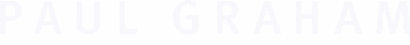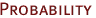Suppose that being over 7 feet tall indicates with 60% probability that someone is a basketball player, and carrying a basketball indicates this with 72% probability. If you see someone who is over 7 feet tall and carrying a basketball, what is the probability that they're a basketball player?If a and b are the probabilities associated with two independent pieces of evidence, then combined they indicate a probability of:``` ab ------------------- ab + (1 - a)(1 - b) ``` So in this case our answer is:``` (.60)(.72) ------------------------------- (.60)(.72) + (1 - .60)(1 - .72) ``` which is .794. There is a 79.4% chance that the person is a basketball player.When there are more than two pieces of evidence, the formula expands as you might expect:``` abc --------------------------- abc + (1 - a)(1 - b)(1 - c) ``` In the case of spam filtering, what we want to calculate is the probability that the mail is a spam, and the individual pieces of evidence a, b, c, ... are the spam probabilities associated with each of the words in the mail.For a good explanation of the background, see:http://www.mathpages.com/home/kmath267.htm.